# Texas Go Math Kindergarten Lesson 7.3 Answer Key Model and Count 13 and 14

Refer to our Texas Go Math Kindergarten Answer Key Pdf to score good marks in the exams. Test yourself by practicing the problems from Texas Go Math Kindergarten Lesson 7.3 Answer Key Model and Count 13 and 14.

## Texas Go Math Kindergarten Lesson 7.3 Answer Key Model and Count 13 and 14

Explore

DIRECTIONS: Use counters to show the number 13. Add more to show the number 14. Draw the counters.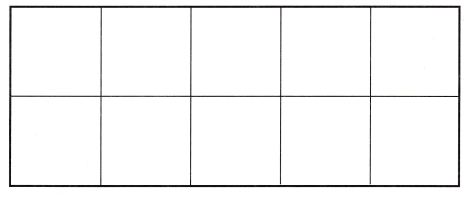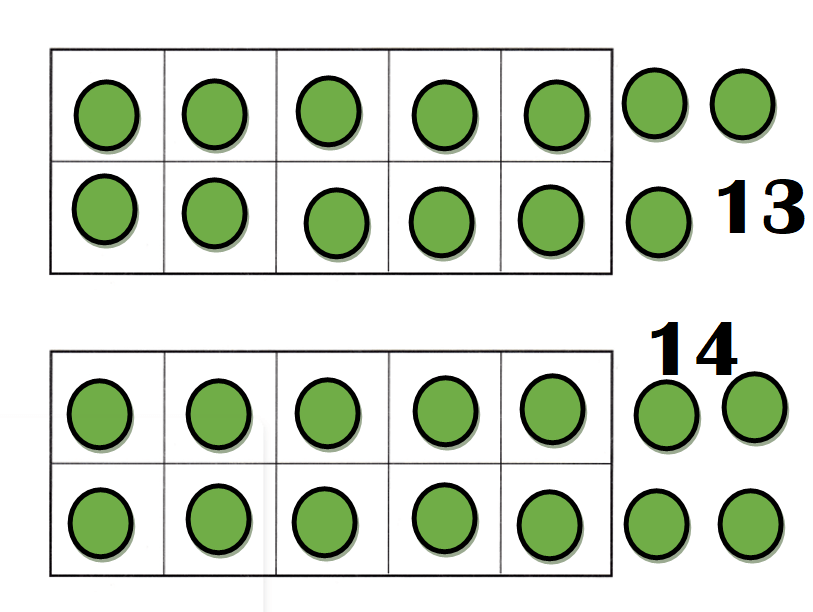Explanation:
Number of counters = 10 + 3 = 13.
Number of counters = 10 + 4 = 14.

Share and Show

DIRECTIONS: 1. Count and tell how many. Circle the number and word. 2. Use counters to show the number 13. Draw the counters. 3. Look at the counters you drew. How many are in the ten frame? Write the number. How many more ore there? Write the number.

Question 1.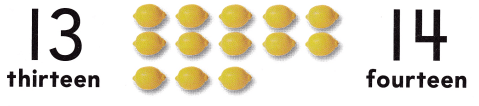Explanation:
Number of lemons = 10 + 3 = 13 or Thirteen.

Question 2.Explanation:
Number of counters = 10 + 3 = 13 or Thirteen.

Question 3.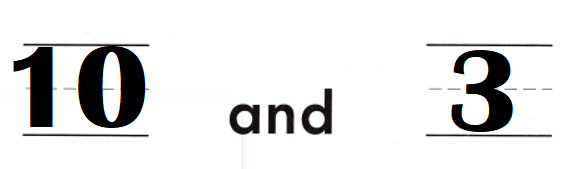Explanation:
Number of counters in the frame = 10.
Number of counters out the frame = 3.
Total number of counters = 10 + 3 = 13 or Thirteen.

DIRECTIONS: 4. Count and tell how many. Circle the number and word. 5. Use counters to show the number 14. Draw the counters. 6. Look at the counters you drew. How many are in the ten frame? Write the number. How many more are there? Write the number.

Question 4.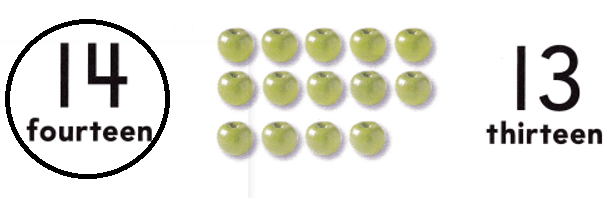Explanation:
Number of apples = 10 + 4 = 14.

Question 5.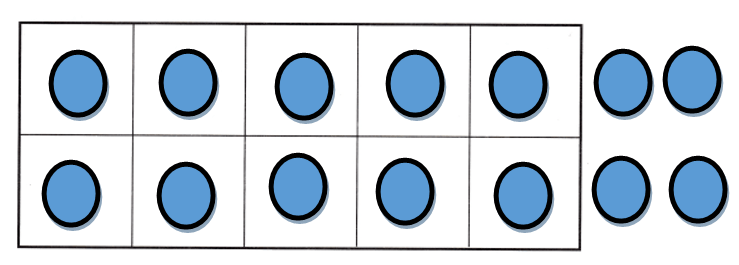Explanation:
Number of counters in frame = 10.
Number of counters out the frame = 4.
Total number of counters = 10 + 4 = 14 or Fourteen.

Question 6.Explanation:
Number of counters in frame = 10.
Number of counters out the frame = 4.
Total number of counters = 10 + 4 = 14 or Fourteen.

Problem Solving
Question 7.Total number of beads = 13 or Thirteen.

Explanation:
Number of  blue beads = 8.
Number of yellow beads = 5.
= 8 + 5
= 13 or Thirteen.

Question 8.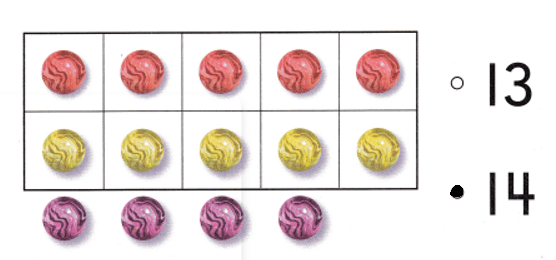Explanation:
Number of marbles = 14 or Fourteen.

### Texas Go Math Kindergarten Lesson 7.3 Homework and Practice Answer Key

DIRECTIONS: 1. Draw counters to show 13. Write the number. 2. Draw counters to show 14. Write the number.

Question 1.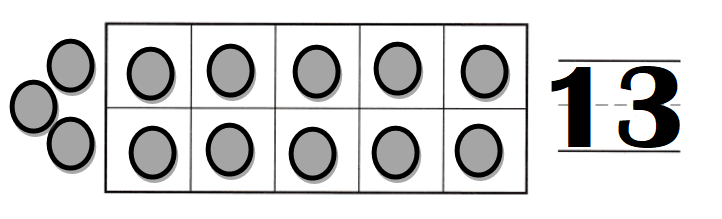Explanation:
Number of counters = 13 or Thirteen.

Question 2.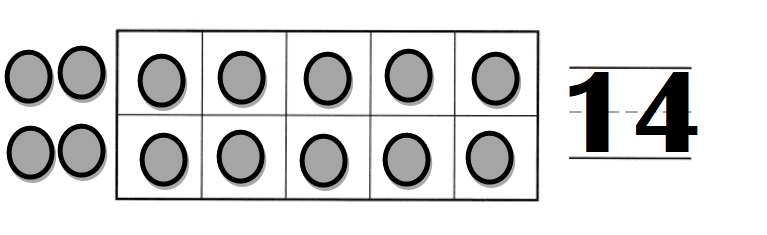Explanation:
Number of counters = 14 or Fourteen.

DIRECTIONS: Choose the correct answer. 3. How many bananas are there? 4. How many pineapples are there? 5. How many oranges are there?

Lesson Check
Question 3.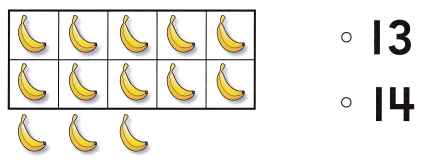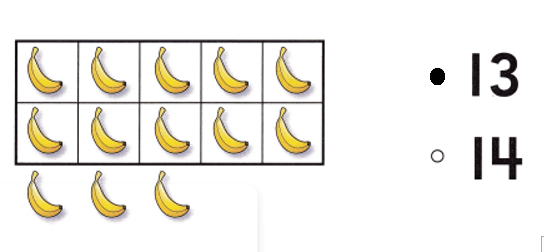Explanation:
Number of bananas = 13 or Thirteen.

Question 4.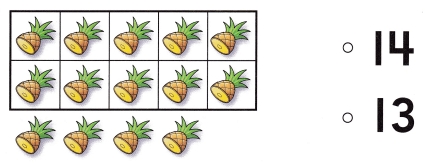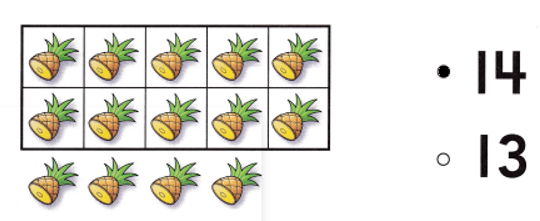Explanation:
Number of Pineapples = 14 or Fourteen.

Question 5.